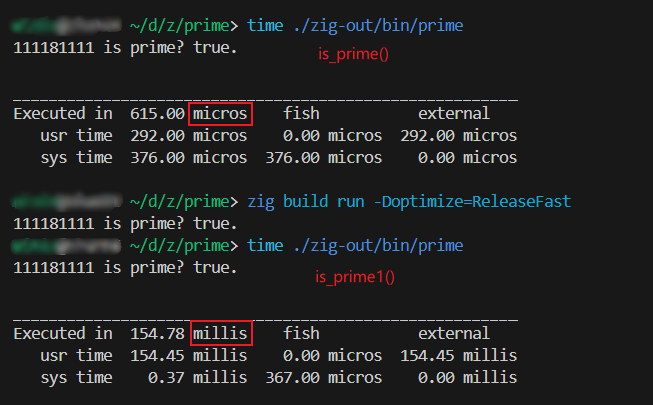# I can't understand the performance difference of my code(Solved,it's not really,my fault.)

my zig version is 0.11.0
there are my codes:

``````const std = @import("std");
const math = std.math;

pub fn main() !void {
const stdout_file = std.io.getStdOut().writer();
var bw = std.io.bufferedWriter(stdout_file);
const stdout = bw.writer();

const n: i32 = 111181111;

try stdout.print("{} is prime? {}.\n", .{ n, is_prime(n) });

try bw.flush(); // don't forget to flush!
}

fn is_prime(n: i32) bool {
var i: i32 = 2;
const f: f64 = @floatFromInt(n);
const floor: i32 = @intFromFloat(math.floor(math.sqrt(f)));
while (i <= floor) {
if (@mod(n, i) == 0) {
return false;
}
i += 1;
}
return true;
}

fn is_prime1(n: i32) bool {
var i: i32 = 2;
const f: f64 = @floatFromInt(n);
const floor: i32 = @intFromFloat(math.floor(math.sqrt(f)));
_ = floor;
while (i < n) {
if (@mod(n, i) == 0) {
return false;
}
i += 1;
}
return true;
}
``````

I don’t know why is_prime() is much slower than is_prime1(), both in debug mode and ReleaseFast mode.In stead, I think is_prime() should have higher performance than is_prime1().

And both in c and go, is_prime() has much better performance than is_prime1().Hi,
Welcome to the Ziggit forum.

Have you looked at the generated assembly using tools like compiler explorer?

For me `is_prime` is a lot faster than `is_prime1`.
`is_prime` took less than 1 ms for the given prime number.

I suspect that you made a measuring error. Is it possible that you timed the compile time as well?
For example running `time zig run test.zig` will measure the compile+execution time. And with the given number the execution time is actually a lot faster than the compile time.

In general I would suggest only benachmarking the part of the code that you actually care about. This ensures that you don’t measure any weird side effects:

``````    const n: i32 = 2000000011;
const t1 = std.time.nanoTimestamp();
const res = is_prime(n);
const t2 = std.time.nanoTimestamp();

try stdout.print("{} is prime? {}.\n Time: {} ns\n", .{ n, res, t2 - t1 });
``````

Now there is one other effect here. Note that `@mod` is not equivalent to `%` in other languages. It behaves differently for negative numbers. And since `@mod` is usually not implemented on the hardware directly(*except from powers of 2), you will loose some performance(unless the compiler figures out that your inputs are positive).
I measured a ~2× performance improvement when replacing `@mod` with `@rem` or when using `u32` instead of `i32`.

1 Like

Oh,I am so sorry that I haven’t noted that these two results were with different unit.You are right.Thanks.This thing was all of my fault.Thank you very much. This problem I said before has not actually happened.I made some mistake. I‘m sorry.

1 Like